# CUDA加速——基于规约思想的数组元素求和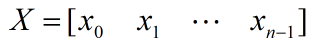X的所有元素和就是：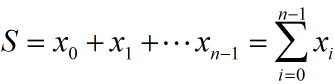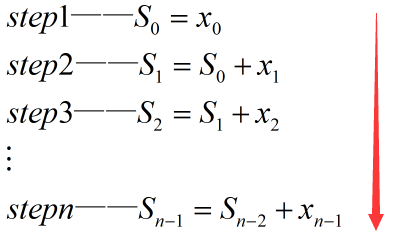# 规约求和思想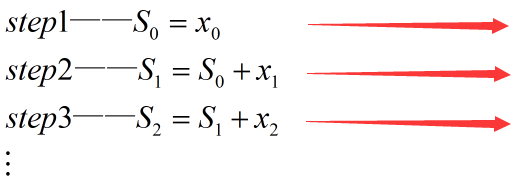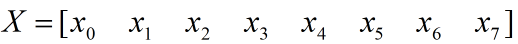• 将x0~x7两两分组：(x0,x1)、(x2,x3)、(x4,x5)、(x6,x7)。然后使用4个线程并行计算每组的元素和，得到4个求和结果：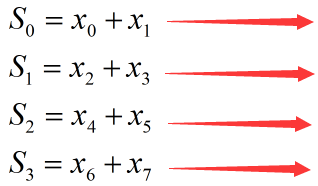• 再将上一步骤得到的4个求和结果两两分组：(S0,S1)、(S2,S3)。然后使用2个线程并行计算每组的元素和，得到2个求和结果：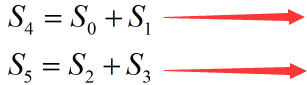• 使用1个线程计算上一步骤得到的S4，S5之和，也即最终求和结果：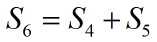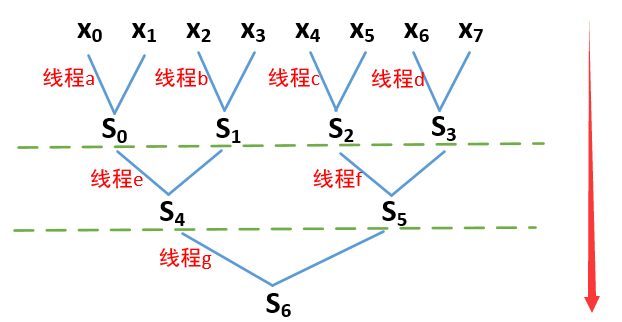# CUDA实现数组元素的规约求和

CUDA是为并行计算而生的，使用CUDA可以很容实现上述的数组规约求和算法。不过有一点需要注意，就是必须确保每个步骤的所有线程是同步的，也即所有线程计算完成之后再进入下一步骤的计算，否则会导致结果错误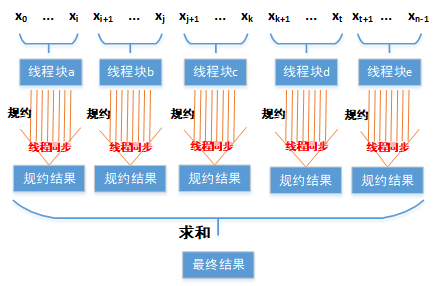CUDA核函数代码如下：

#define N (1536 * 20480)   //数据总长度

//Para为输入数组，长度为N
//blocksum_cuda存储所有线程块的规约结果
__global__ void cal_sum_ker0(float *Para, float *blocksum_cuda)
{
//计算线程ID号
//blockIdx.x为线程块的ID号
//blockDim.x每个线程块中包含的线程总个数
int tid = blockIdx.x * blockDim.x + threadIdx.x;

if(tid < N)
{
for (int index = 1; index < blockDim.x; index = (index*2))
{
if (threadIdx.x % (index*2) == 0)
{
Para[tid] += Para[tid + index];  //规约求和
}

}

blocksum_cuda[blockIdx.x] = Para[tid];
}
}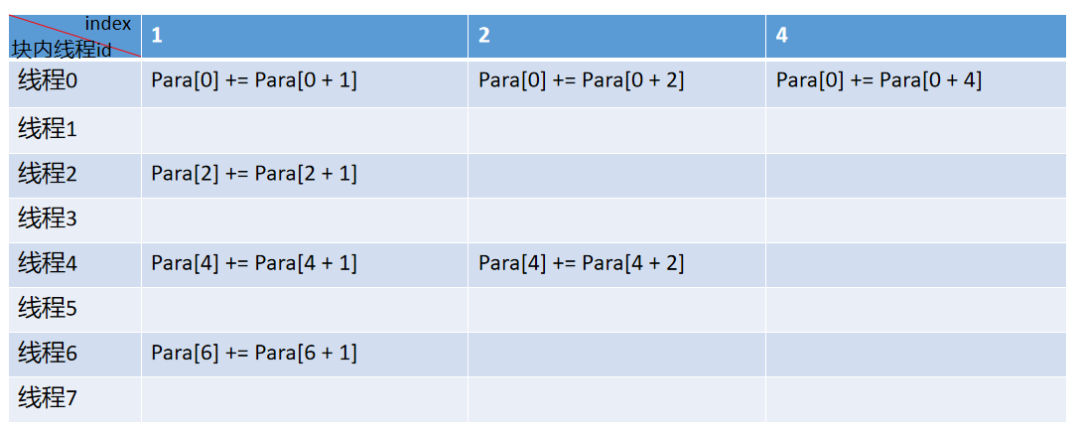class Timer_Us2
{
private:
LARGE_INTEGER cpuFreq;
LARGE_INTEGER startTime;
LARGE_INTEGER endTime;
public:
double rumTime;
void get_frequence(void)
{
QueryPerformanceFrequency(&cpuFreq);   //获取时钟频率
}

void start_timer(void)
{
QueryPerformanceCounter(&startTime);    //开始计时
}

void stop_timer(char *str)
{
QueryPerformanceCounter(&endTime);    //结束计时
cout << str << rumTime << " ms" << endl;
}

Timer_Us2()    //构造函数
{
QueryPerformanceFrequency(&cpuFreq);
}
};

void Cal_Sum_Test()
{
Timer_Us2 timer;
//申请长度为N的float型动态内存
float *test_d = (float *)malloc(N * sizeof(float));
for (long long i = 0; i < N; i++)
{
test_d[i] = 0.5;  //将所有元素赋值为0.5
}

double ParaSum = 0.0;

timer.start_timer();
//在CPU端按顺序计算数组元素和
for (long long i = 0; i < N; i++)
{
ParaSum += test_d[i];  //CPU端数组累加
}
timer.stop_timer("CPU time:");
cout << " CPU result = " << ParaSum << endl;  //显示CPU端结果

//设置每个线程块有1024个线程
dim3 sumblock(1024);
//设置总共有多少个线程块
dim3 sumgrid(((N%sumblock.x) ? (N/sumblock.x + 1) : (N/sumblock.x)));

float *test_d_cuda;
float *blocksum_cuda;
float *blocksum_host = (float *)malloc(sizeof(float) * sumgrid.x);
//申请GPU端全局内存
cudaMalloc((void **)&test_d_cuda, sizeof(float) * N);
cudaMalloc((void **)&blocksum_cuda, sizeof(float) * sumgrid.x);

timer.start_timer();
//将数据从CPU端拷贝到GPU端
cudaMemcpy(test_d_cuda, test_d, sizeof(float) * N, cudaMemcpyHostToDevice);
//调用核函数进行规约求和
cal_sum_ker0 << < sumgrid, sumblock >> > (test_d_cuda, blocksum_cuda);
//将每个线程块的规约求和结果拷贝到CPU端
cudaMemcpy(blocksum_host, blocksum_cuda, sizeof(float) * sumgrid.x, cudaMemcpyDeviceToHost);
//在CPU端对所有线程块的规约求和结果做串行求和
double sum = 0.0;
for(int i = 0; i < sumgrid.x; i++)
{
sum += blocksum_host[i];
}

timer.stop_timer("GPU time:");

cout << " GPU result = " << sum << endl;   //显示GPU端结果

//释放内存
cudaFree(test_d_cuda);
cudaFree(blocksum_cuda);
free(blocksum_host);
free(test_d);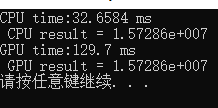10-15317
03-17

03-03402
10-04198
09-231337
05-16253
12-11453
11-19775
10-015443
03-082024
10-186326
10-116482
04-283115
04-284350
12-17599
09-291439
05-1767
12-25280
11-19938

### “相关推荐”对你有帮助么？

•非常没帮助
•没帮助
•一般
•有帮助
•非常有帮助

©️2022 CSDN 皮肤主题：Age of Ai 设计师：meimeiellie¥2 ¥4 ¥6 ¥10 ¥20余额支付 (余额：-- )扫码支付获取中扫码支付点击重新获取扫码支付1.余额是钱包充值的虚拟货币，按照1:1的比例进行支付金额的抵扣。
2.余额无法直接购买下载，可以购买VIP、C币套餐、付费专栏及课程。余额充值